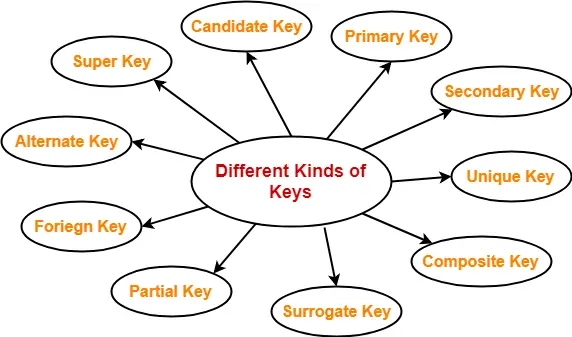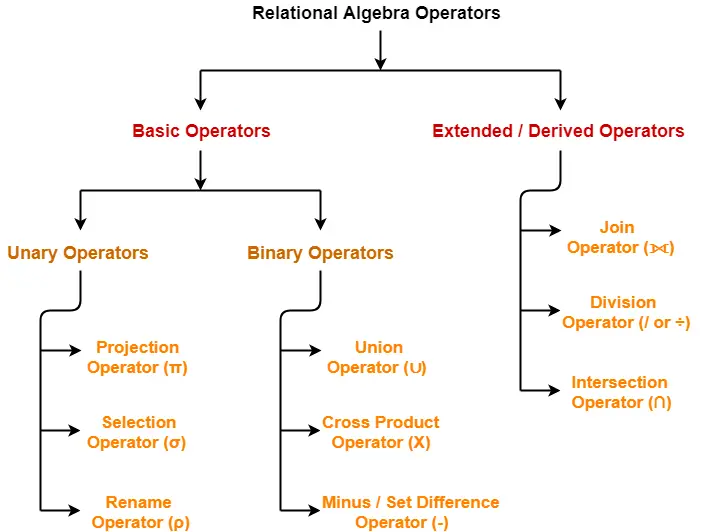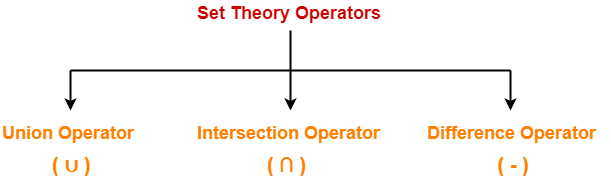## Keys in DBMS-

Before you go through this article, make sure that you have gone through the previous article on Keys in DBMS.

We have discussed-

• A key is a set of attributes that can identify each tuple uniquely in the given relation.
• There are various types of keys in DBMS-In this article, we will discuss how to find candidate keys of a given relation.

## Candidate Key-

A candidate key may be defined as-

 A set of minimal attribute(s) that can identify each tuple uniquely in the given relation is called as a candidate key. OR A minimal super key is called as a candidate key.

For any given relation,

• It is possible to have multiple candidate keys.
• There exists no general formula to find the total number of candidate keys of a given relation.

## Example-

Consider the following Student schema-

Student ( roll , name , sex , age , address , class , section )

Given below are the examples of candidate keys-

• ( class , section , roll )
• ( name , address )

These are candidate keys because each set consists of minimal attributes required to identify each student uniquely in the Student table.

## Finding Candidate Keys-

We can determine the candidate keys of a given relation using the following steps-

### Step-01:

• Determine all essential attributes of the given relation.
• Essential attributes are those attributes which are not present on RHS of any functional dependency.
• Essential attributes are always a part of every candidate key.
• This is because they can not be determined by other attributes.

### Example

Let R(A, B, C, D, E, F) be a relation scheme with the following functional dependencies-

A → B

C → D

D → E

Here, the attributes which are not present on RHS of any functional dependency are A, C and F.

So, essential attributes are- A, C and F.

### Step-02:

• The remaining attributes of the relation are non-essential attributes.
• This is because they can be determined by using essential attributes.

Now, following two cases are possible-

### Case-01:

If all essential attributes together can determine all remaining non-essential attributes, then-

• The combination of essential attributes is the candidate key.
• It is the only possible candidate key.

### Case-02:

If all essential attributes together can not determine all remaining non-essential attributes, then-

• The set of essential attributes and some non-essential attributes will be the candidate key(s).
• In this case, multiple candidate keys are possible.
• To find the candidate keys, we check different combinations of essential and non-essential attributes.

We will further understand how to find candidate keys with the help of following problems.

The following practice problems are based on Case-01.

## Problem-01:

Let R = (A, B, C, D, E, F) be a relation scheme with the following dependencies-

C → F

E → A

EC → D

A → B

Which of the following is a key for R?

1. CD
2. EC
3. AE
4. AC

Also, determine the total number of candidate keys and super keys.

## Solution-

We will find candidate keys of the given relation in the following steps-

### Step-01:

• Determine all essential attributes of the given relation.
• Essential attributes of the relation are- C and E.
• So, attributes C and E will definitely be a part of every candidate key.

Step-02:

Now,

• We will check if the essential attributes together can determine all remaining non-essential attributes.
• To check, we find the closure of CE.

So, we have-

{ CE }+

= { C , E }

= { C , E , F } ( Using C → F )

= { A , C , E , F } ( Using E → A )

= { A , C , D , E , F } ( Using EC → D )

= { A , B , C , D , E , F } ( Using A → B )

We conclude that CE can determine all the attributes of the given relation.

So, CE is the only possible candidate key of the relation.

Thus, Option (B) is correct.

### Total Number of Candidate Keys-

Only one candidate key CE is possible.

### Total Number of Super Keys-

There are total 6 attributes in the given relation of which-

• There are 2 essential attributes- C and E.
• Remaining 4 attributes are non-essential attributes.
• Essential attributes will be definitely present in every key.
• Non-essential attributes may or may not be taken in every super key.So, number of super keys possible = 2 x 2 x 2 x 2 = 16.

Thus, total number of super keys possible = 16.

## Problem-02:

Let R = (A, B, C, D, E) be a relation scheme with the following dependencies-

AB → C

C → D

B → E

Determine the total number of candidate keys and super keys.

## Solution-

We will find candidate keys of the given relation in the following steps-

### Step-01:

• Determine all essential attributes of the given relation.
• Essential attributes of the relation are- A and B.
• So, attributes A and B will definitely be a part of every candidate key.

Step-02:

Now,

• We will check if the essential attributes together can determine all remaining non-essential attributes.
• To check, we find the closure of AB.

So, we have-

{ AB }+

= { A , B }

= { A , B , C } ( Using AB → C )

= { A , B , C , D } ( Using C → D )

= { A , B , C , D , E } ( Using B → E )

We conclude that AB can determine all the attributes of the given relation.

Thus, AB is the only possible candidate key of the relation.

### Total Number of Candidate Keys-

Only one candidate key AB is possible.

### Total Number of Super Keys-

There are total 5 attributes in the given relation of which-

• There are 2 essential attributes- A and B.
• Remaining 3 attributes are non-essential attributes.
• Essential attributes will be definitely present in every key.
• Non-essential attributes may or may not be taken in every super key.So, number of super keys possible = 2 x 2 x 2 = 8.

Thus, total number of super keys possible = 8.

## Problem-03:

Consider the relation scheme R(E, F, G, H, I, J, K, L, M, N) and the set of functional dependencies-

{ E, F } → { G }

{ F } → { I , J }

{ E, H } → { K, L }

{ K } → { M }

{ L } → { N }

What is the key for R?

1. { E, F }
2. { E, F, H }
3. { E, F, H, K, L }
4. { E }

Also, determine the total number of candidate keys and super keys.

## Solution-

We will find candidate keys of the given relation in the following steps-

### Step-01:

• Determine all essential attributes of the given relation.
• Essential attributes of the relation are- E, F and H.
• So, attributes E, F and H will definitely be a part of every candidate key.

Step-02:

Now,

• We will check if the essential attributes together can determine all remaining non-essential attributes.
• To check, we find the closure of EFH.

So, we have-

{ EFH }+

= { E , F , H }

= { E , F , G , H } ( Using EF → G )

= { E , F , G , H , I , J } ( Using F → IJ )

= { E , F , G , H , I , J , K , L } ( Using EH → KL )

= { E , F , G , H , I , J , K , L , M } ( Using K → M )

= { E , F , G , H , I , J , K , L , M , N } ( Using L → N )

We conclude that EFH can determine all the attributes of the given relation.

So, EFH is the only possible candidate key of the relation.

Thus, Option (B) is correct.

### Total Number of Candidate Keys-

Only one candidate key EFH is possible.

### Total Number of Super Keys-

There are total 10 attributes in the given relation of which-

• There are 3 essential attributes- E, F and H.
• Remaining 7 attributes are non-essential attributes.
• Essential attributes will be definitely present in every key.
• Non-essential attributes may or may not be taken in every super key.So, number of super keys possible = 2 x 2 x 2 x 2 x 2 x 2 x 2 = 128.

Thus, total number of super keys possible = 128.

## Problem-04:

Consider the relation scheme R(A, B, C, D, E, H) and the set of functional dependencies-

A → B

BC → D

E → C

D → A

What are the candidate keys of R?

1. AE, BE
2. AE, BE, DE
3. AEH, BEH, BCH
4. AEH, BEH, DEH

## Solution-

We will find candidate keys of the given relation in the following steps-

### Step-01:

• Determine all essential attributes of the given relation.
• Essential attributes of the relation are- E and H.
• So, attributes E and H will definitely be a part of every candidate key.

The only possible option is (D).

Thus, Option (D) is correct.

Next Article- Functional Dependency in DBMS

Get more notes and other study material of Database Management System (DBMS).

Watch video lectures by visiting our YouTube channel LearnVidFun.

## Relational Algebra Operators-

Before you go through this article, make sure that you have gone through the previous article on Introduction to Relational Algebra.

The operators in relational algebra are classified as-## Set Theory Operators-

Following operators are called as set theory operators-1. Union Operator (∪)
2. Intersection Operator (∩)
3. Difference Operator (-)

### Condition For Using Set Theory Operators

To use set theory operators on two relations,

The two relations must be union compatible.

Union compatible property means-

• Both the relations must have same number of attributes.
• The attribute domains (types of values accepted by attributes) of both the relations must be compatible.

Also read- Selection Operator and Projection Operator

## 1. Union Operator (∪)-

Let R and S be two relations.

Then-

• R ∪ S is the set of all tuples belonging to either R or S or both.
• In R ∪ S, duplicates are automatically removed.
• Union operation is both commutative and associative.

## Example-

Consider the following two relations R and S-

 ID Name Subject 100 Ankit English 200 Pooja Maths 300 Komal Science

#### Relation R

 ID Name Subject 100 Ankit English 400 Kajol French

#### Relation S

Then, R ∪ S is-

 ID Name Subject 100 Ankit English 200 Pooja Maths 300 Komal Science 400 Kajol French

## 2. Intersection Operator (∩)-

Let R and S be two relations.

Then-

• R ∩ S is the set of all tuples belonging to both R and S.
• In R ∩ S, duplicates are automatically removed.
• Intersection operation is both commutative and associative.

## Example-

Consider the following two relations R and S-

 ID Name Subject 100 Ankit English 200 Pooja Maths 300 Komal Science

#### Relation R

 ID Name Subject 100 Ankit English 400 Kajol French

#### Relation S

Then, R ∩ S is-

 ID Name Subject 100 Ankit English

## 3. Difference Operator (-)-

Let R and S be two relations.

Then-

• R – S is the set of all tuples belonging to R and not to S.
• In R – S, duplicates are automatically removed.
• Difference operation is associative but not commutative.

### Example-

Consider the following two relations R and S-

 ID Name Subject 100 Ankit English 200 Pooja Maths 300 Komal Science

#### Relation R

 ID Name Subject 100 Ankit English 400 Kajol French

#### Relation S

Then, R – S is-

 ID Name Subject 200 Pooja Maths 300 Komal Science

#### Relation R – S

Get more notes and other study material of Database Management System (DBMS).

Watch video lectures by visiting our YouTube channel LearnVidFun.

## Relational Algebra Operators-

Before you go through this article, make sure that you have gone through the previous article on Introduction to Relational Algebra.

The operators in relational algebra are classified as-## Projection Operator-

• Projection Operator (π) is a unary operator in relational algebra that performs a projection operation.
• It displays the columns of a relation or table based on the specified attributes.

 π(R)

## Example-

Consider the following Student relation-

 ID Name Subject Age 100 Ashish Maths 19 200 Rahul Science 20 300 Naina Physics 20 400 Sameer Chemistry 21

Then, we have-

### Result for Query πName, Age(Student)-

 Name Age Ashish 19 Rahul 20 Naina 20 Sameer 21

### Result for Query πID , Name(Student)-

 ID Name 100 Ashish 200 Rahul 300 Naina 400 Sameer

## Point-01:

• The degree of output relation (number of columns present) is equal to the number of attributes mentioned in the attribute list.

## Point-02:

• Projection operator automatically removes all the duplicates while projecting the output relation.
• So, cardinality of the original relation and output relation may or may not be same.
• If there are no duplicates in the original relation, then the cardinality will remain same otherwise it will surely reduce.

## Point-03:

• If attribute list is a super key on relation R, then we will always get the same number of tuples in the output relation.
• This is because then there will be no duplicates to filter.

## Point-04:

• Projection operator does not obey commutative property i.e.

π <list2><list1> (R)) ≠ π <list1><list2> (R))

## Point-05:

• Following expressions are equivalent because both finally projects columns of list-1

π <list1><list2> (R)) = π <list1> (R)

## Point-06:

• Selection Operator performs horizontal partitioning of the relation.
• Projection operator performs vertical partitioning of the relation.

## Point-07:

• There is only one difference between projection operator of relational algebra and SELECT operation of SQL.
• Projection operator does not allow duplicates while SELECT operation allows duplicates.
• To avoid duplicates in SQL, we use “distinct” keyword and write SELECT distinct.
• Thus, projection operator of relational algebra is equivalent to SELECT operation of SQL.

Next Article- Set Theory Operators in Relational Algebra

Get more notes and other study material of Database Management System (DBMS).

Watch video lectures by visiting our YouTube channel LearnVidFun.

## Relational Algebra Operators-

Before you go through this article, make sure that you have gone through the previous article on Introduction to Relational Algebra.

The operators in relational algebra are classified as-## Selection Operator-

• Selection Operator (σ) is a unary operator in relational algebra that performs a selection operation.
• It selects those rows or tuples from the relation that satisfies the selection condition.

 σ(R)

## Examples-

• Select tuples from a relation “Books” where subject is “database”

σsubject = “database” (Books)

• Select tuples from a relation “Books” where subject is “database” and price is “450”

σsubject = “database” ∧ price = “450” (Books)

• Select tuples from a relation “Books” where subject is “database” and price is “450” or have a publication year after 2010

σsubject = “database” ∧ price = “450” ∨ year >”2010″ (Books)

## Point-01:

• We may use logical operators like ∧ , ∨ , ! and relational operators like = , ≠ , > , < , <= , >= with the selection condition.

## Point-02:

• Selection operator only selects the required tuples according to the selection condition.
• It does not display the selected tuples.
• To display the selected tuples, projection operator is used.

## Point-03:

• Selection operator always selects the entire tuple. It can not select a section or part of a tuple.

## Point-04:

• Selection operator is commutative in nature i.e.

σ A ∧ B (R) = σ B ∧ A (R)

OR

σ B A(R)) = σ A B(R))

## Point-05:

• Degree of the relation from a selection operation is same as degree of the input relation.

## Point-06:

• The number of rows returned by a selection operation is obviously less than or equal to the number of rows in the original table.

Thus,

• Minimum Cardinality = 0
• Maximum Cardinality = |R|

Next Article- Projection Operation in Relational Algebra

Get more notes and other study material of Database Management System (DBMS).

Watch video lectures by visiting our YouTube channel LearnVidFun.

## Relational Algebra-

 Relational Algebra is a procedural query language which takes a relation as an input and generates a relation as an output.

## Relational Algebra Operators-

The operators in relational algebra may be classified as-We will discuss all these operators one by one in detail.

## Characteristics-

Following are the important characteristics of relational operators-

• Relational Operators always work on one or more relational tables.
• Relational Operators always produce another relational table.
• The table produced by a relational operator has all the properties of a relational model.

Next Article- Selection Operator in Relational Algebra

Get more notes and other study material of Database Management System (DBMS).

Watch video lectures by visiting our YouTube channel LearnVidFun.